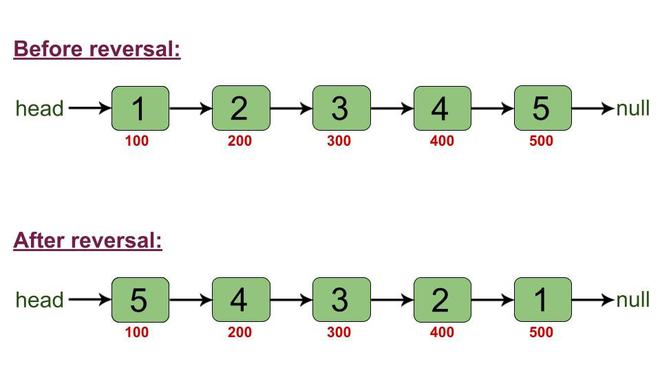# Java Program to Reverse a Linked List Without Manipulating its Pointers

• Difficulty Level : Medium
• Last Updated : 24 Feb, 2021

Given a linked list, the task is to write a program in Java that reverses the linked list without manipulating its pointers, i.e., the reversal should happen just by changing the data values and not the links.

Examples:

Attention reader! Don’t stop learning now. Get hold of all the important Java Foundation and Collections concepts with the Fundamentals of Java and Java Collections Course at a student-friendly price and become industry ready. To complete your preparation from learning a language to DS Algo and many more,  please refer Complete Interview Preparation Course.

```Input: Original linked list
1->2->3->4->5->null
5->4->3->2->1->null

5->14->20->8->null
8->20->14->5->null

80->null
80->null```

For reversal, no manipulation is done in the links that connect the node of the linked list. Only the data values are changed. To understand this more clearly, take a look at the following diagram.The numbers in red color represent the addresses of nodes. It is to be noticed that even after the reversal, the links remained the same, i.e., before reversing, the node at address 100 was connected to the node at address 200, which in turn was connected to the node at address 300 and so on, and these connections have remained the same after the reversal as well.

Approach:

1. Variables ‘l’ and ‘r’ are initialized as 0 and size-1 respectively, denoting the index of first and last node.
2. In a loop, the nodes at index ‘l’ and ‘r’ are obtained and the corresponding data values are swapped. The loop works by incrementing ‘l’ and decrementing ‘r’.
3. For getting the node at a particular index, a private method ‘fetchNode’ is defined.

Below is the implementation of the above approach:

## Java

 `// Java program to reverse a linked list without pointer``// manipulation` `class` `Node {``    ``int` `value;``    ``Node next;` `    ``Node(``int` `val)``    ``{``        ``value = val;``        ``next = ``null``;``    ``}``}` `public` `class` `LinkedList {``    ``Node head;` `    ``// this function returns the Node which is at a``    ``// particular index.``    ``// (The index is passed as the argument)``    ``private` `Node fetchNode(``int` `index)``    ``{``        ``Node temp = head;``        ``for` `(``int` `i = ``0``; i < index; i++) {``            ``temp = temp.next;``        ``}``        ``return` `temp;``    ``}` `    ``// this function returns the size of linked list``    ``int` `getSize(Node head)``    ``{``        ``Node temp = head;``        ``int` `size = ``0``;``        ``while` `(temp != ``null``) {``            ``size++;``            ``temp = temp.next;``        ``}``        ``return` `size;``    ``}` `    ``// function to reverse the linked list``    ``void` `reverse()``    ``{``        ``int` `l = ``0``;``        ``int` `r = getSize(``this``.head) - ``1``;``        ``while` `(l < r) {``            ``Node leftSideNode = fetchNode(l);``            ``Node rightSideNode = fetchNode(r);` `            ``int` `t = leftSideNode.value;``            ``leftSideNode.value = rightSideNode.value;``            ``rightSideNode.value = t;` `            ``l++;``            ``r--;``        ``}``    ``}` `    ``// function that prints the elements of linked list``    ``void` `printLinkedList()``    ``{``        ``Node temp = ``this``.head;``        ``while` `(temp != ``null``) {``            ``System.out.print(temp.value + ``" "``);``            ``temp = temp.next;``        ``}``        ``System.out.println();``    ``}` `    ``// Driver code``    ``public` `static` `void` `main(String[] args)``    ``{``        ``LinkedList list1 = ``new` `LinkedList();``        ``list1.head = ``new` `Node(``1``);``        ``list1.head.next = ``new` `Node(``2``);``        ``list1.head.next.next = ``new` `Node(``3``);``        ``list1.head.next.next.next = ``new` `Node(``4``);``        ``list1.head.next.next.next.next = ``new` `Node(``5``);` `        ``System.out.println(``"Linked List Before Reversal: "``);``        ``list1.printLinkedList();` `        ``list1.reverse();` `        ``System.out.println(``"Linked List After Reversal: "``);``        ``list1.printLinkedList();``    ``}``}`

Output
```Linked List Before Reversal:
1 2 3 4 5